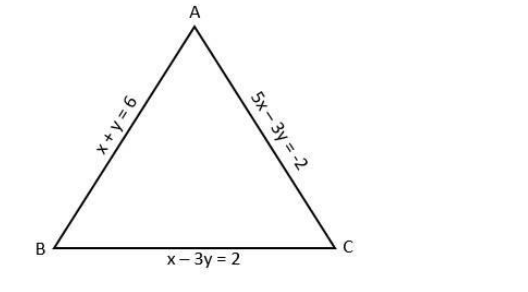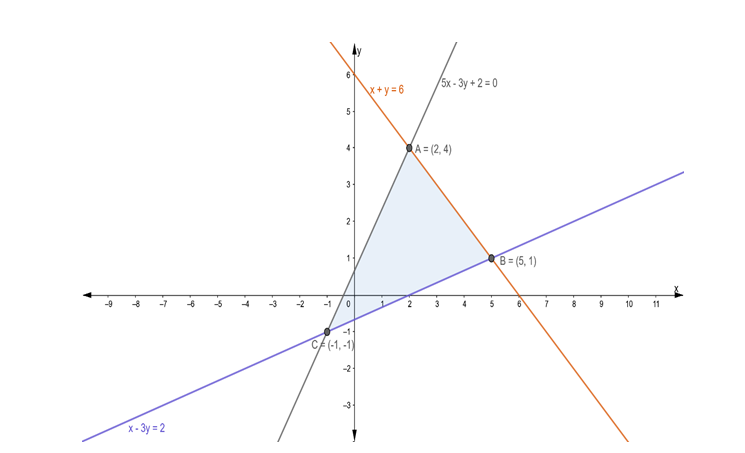# Find the area of the triangle formed by the lines`
Question:

Find the area of the triangle formed by the lines x + y = 6, x – 3y = 2 and 5x – 3y + 2 = 0.

Solution:

The given equations are

$x+y=6 \ldots(i)$

$x-3 y=2 \ldots(i i)$

and $5 x-3 y+2=0$

or $5 x-3 y=-2 \ldots$ (iii)

Let eq. (i), (ii) and (iii) represents the sides AB, BC and AC respectively of ΔABCFirstly, we solve the equation (i) and (ii)

$x+y=6 \ldots(i)$

$x-3 y=2 \ldots(i i)$

Subtracting eq. (ii) from (i), we get

$x+y-x+3 y=6-2$

$\Rightarrow 4 y=4$

$\Rightarrow y=1$

Putting the value of y = 1 in eq. (i), we get

$x+1=6$

$\Rightarrow x=5$

Thus, AB and BC intersect at (5, 1)

Now, we solve eq. (ii) and (iii)

$x-3 y=2 \ldots$ (ii)

$5 x-3 y=-2 \ldots$ (ii)

Subtracting eq. (ii) from (iii), we get

$5 x-3 y-x+3 y=-2-2$

$\Rightarrow 4 x=-4$

$\Rightarrow x=-1$

Putting the value of $x=-1$ in eq. (ii), we get

$-1-3 y=2$

$\Rightarrow-3 y=2+1$

$\Rightarrow-3 y=3$

$\Rightarrow y=-1$

Thus, $B C$ and $A C$ intersect at $(-1,-1)$

Now, we solve eq. (iii) and (i)

$5 x-3 y=-2 \ldots$ (iii)

$x+y=6 \ldots$ (i)

From eq. (i), we get

$x=6-y$

Putting the value of x in eq. (iii), we get

$5(6-y)-3 y=-2$

$\Rightarrow 30-5 y-3 y=-2$

$\Rightarrow 30-8 y=-2$

$\Rightarrow-8 y=-32$

$\Rightarrow y=4$

Putting the value of y = 4 in eq. (i), we get

$x+4=6$

$\Rightarrow x=6-4$

$\Rightarrow x=2$

Thus, $A C$ and $A B$ intersect at $(2,4)$

So, vertices of triangle $A B C$ are: $(5,1),(-1,-1)$ and $(2,4)$$\therefore$ Area of $\Delta \mathrm{ABC}=\frac{1}{2}\left|\begin{array}{ccc}2 & 4 & 1 \\ 5 & 1 & 1 \\ -1 & -1 & 1\end{array}\right|$

$=\frac{1}{2}[2\{(1)(1)-(1)(-1)\}-4\{(5)(1)-(1)(-1)\}+1\{(5)(-1)-(1)(-1)\}]$

$=\frac{1}{2}[2\{1+1\}-4\{5+1\}+1\{-5+1\}]$

$=\frac{1}{2}[4-24-4]$

$=\frac{1}{2}[|-24|]$

$=12$ sq. units [ $\because$, area can't be negative]